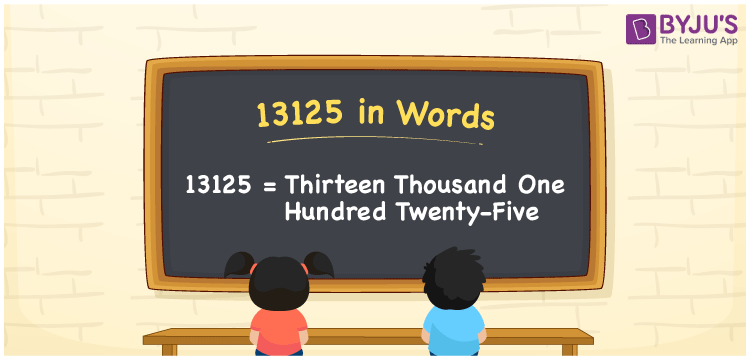# 13125 in Words

We can write 13125 in words as Thirteen thousand one hundred twenty-five. If you paid Rs. 13125 to buy a new Dining table, you could say, “I paid Thirteen thousand one hundred twenty-five rupees for the new Dining table”. Also, we know that 13125 is a cardinal number since it denotes a specific amount or quantity.

 13125 in words Thirteen thousand one hundred twenty-five Thirteen thousand one hundred twenty-five in Numbers 13125

## 13125 in English Words

Generally, we use the English alphabet to write numbers in words. Thus, we can spell 13125 in English words as “Thirteen thousand one hundred twenty-five”.## How to Write 13125 in Words?

Let us understand how to convert 13125 into word form using a five-column place value chart since 13125 contains five digits.

 Ten thousands Thousands Hundreds Tens Ones 1 3 1 2 5

Here, ones = 5, tens = 2, hundreds = 1, thousands = 3, ten thousands = 1

Thus, 1 × Ten thousand + 3 × Thousand + 1 × Hundred + 2 × Ten + 5 × One

= 1 × 10000 + 3 × 1000 + 1 × 100 + 2 × 10 + 5 × 1

= 10000 + 3000 + 100 + 20 + 5

= Ten Thousand + Three thousand + One hundred + Twenty + Five

= Thirteen thousand + One hundred twenty-five {since ten + three = thirteen}

= Thirteen thousand one hundred twenty-five

Therefore, 13125 in words = Thirteen thousand one hundred twenty-five

13125 is a natural number that is the successor of 13124 and predecessor of 13126.

13125 in words – Thirteen thousand one hundred twenty-five

Is 13125 an odd number? – Yes

Is 13125 an even number? – No

Is 13125 a composite number? – Yes

Is 13125 a prime number? – No

Is 13125 a perfect square number? – No

Is 13125 a perfect cube number? – No

## Frequently Asked Questions on 13125 in Words

Q1

### How do you write 13125 in words?

We can write the number 13125 in words as Thirteen thousand one hundred twenty-five.
Q2

### How to write Rs. 13125 in words on a cheque?

On a cheque, we can write an amount of Rs. 13125 in words as “Thirteen thousand one hundred twenty-five rupees only”.
Q3

### Express the value of 13125 – 11813 in words.

13125 – 11813 = 1312 Therefore, the value 13125 – 11813, i.e., 1312 can be expressed in words as One thousand three hundred and twelve.